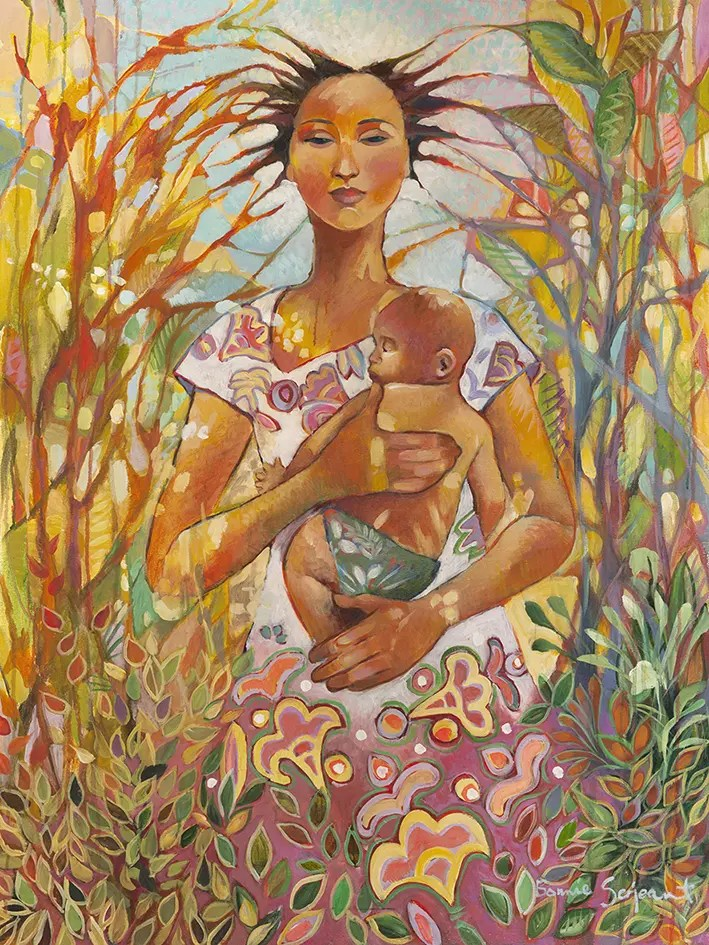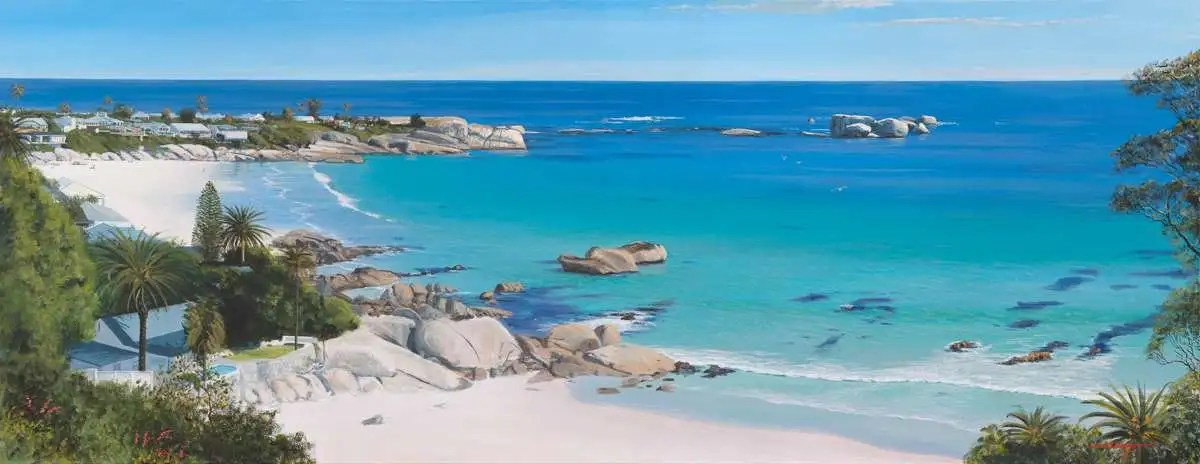##### Currency Conversion from ZAR to:
[convert number=35000.00 from="zar" to="usd"] USD | [convert number=35000.00 from="zar" to="gbp"] GBP | [convert number=35000.00 from="zar" to="eur"] EUR
Artist: [popup_anything id="429762"]

Medium: Acrylic on Canvas

Size: 120cm by 90cm

R 35,000.00##### Currency Conversion from ZAR to:
[convert number=10000.00 from="zar" to="usd"] USD | [convert number=10000.00 from="zar" to="gbp"] GBP | [convert number=10000.00 from="zar" to="eur"] EUR
Artist: [popup_anything id="494114"]

Medium: Acrylic on Canvas

Size: 105cm by 77cm

R 10,000.00##### Currency Conversion from ZAR to:
[convert number=28500.00 from="zar" to="usd"] USD | [convert number=28500.00 from="zar" to="gbp"] GBP | [convert number=28500.00 from="zar" to="eur"] EUR
Artist: [popup_anything id="336014"]
Medium: Acrylic on Canvas
Size: 102cm by 76cm

R 28,500.00##### Currency Conversion from ZAR to:
[convert number=26500.00 from="zar" to="usd"] USD | [convert number=26500.00 from="zar" to="gbp"] GBP | [convert number=26500.00 from="zar" to="eur"] EUR
Artist: [popup_anything id="336014"]
Medium: Acrylic on Canvas
Size: 87cm by 76cm

R 26,500.00##### Currency Conversion from ZAR to:
[convert number=37000.00 from="zar" to="usd"] USD | [convert number=37000.00 from="zar" to="gbp"] GBP | [convert number=37000.00 from="zar" to="eur"] EUR
Artist: [popup_anything id="336020"] Medium: Acrylic on Canvas Size: 196,5cm by 148cm SOLD##### Currency Conversion from ZAR to:
[convert number=28000 from="zar" to="usd"] USD | [convert number=28000 from="zar" to="gbp"] GBP | [convert number=28000 from="zar" to="eur"] EUR
Artist: [popup_anything id="429972"]

Medium: Acrylic on Canvas

Size: 40cm by 28cm

R 28,000.00##### Currency Conversion from ZAR to:
[convert number=28000 from="zar" to="usd"] USD | [convert number=28000 from="zar" to="gbp"] GBP | [convert number=28000 from="zar" to="eur"] EUR
Artist: [popup_anything id="429972"]

Medium: Acrylic on Canvas

Size: 40cm by 28cm

R 28,000.00##### Currency Conversion from ZAR to:
[convert number=35000 from="zar" to="usd"] USD | [convert number=35000 from="zar" to="gbp"] GBP | [convert number=35000 from="zar" to="eur"] EUR
Artist: [popup_anything id="429972"]

Medium: Acrylic on Canvas

Size: 40cm by 60cm

R 35,000.00##### Currency Conversion from ZAR to:
[convert number=55000 from="zar" to="usd"] USD | [convert number=55000 from="zar" to="gbp"] GBP | [convert number=55000 from="zar" to="eur"] EUR
Artist: [popup_anything id="429972"]

Medium: Acrylic on Canvas

Size: 75cm by 45cm

R 55,000.00##### Currency Conversion from ZAR to:
[convert number=65000 from="zar" to="usd"] USD | [convert number=65000 from="zar" to="gbp"] GBP | [convert number=65000 from="zar" to="eur"] EUR
Artist: [popup_anything id="429972"]

Medium: Acrylic on Canvas

Size: 50cm by 80cm

R 65,000.00##### Currency Conversion from ZAR to:
[convert number=85000 from="zar" to="usd"] USD | [convert number=85000 from="zar" to="gbp"] GBP | [convert number=85000 from="zar" to="eur"] EUR
Artist: [popup_anything id="429972"]

Medium: Acrylic on Canvas

Size: 90cm by 80cm

R 85,000.00##### Currency Conversion from ZAR to:
[convert number=90000 from="zar" to="usd"] USD | [convert number=90000 from="zar" to="gbp"] GBP | [convert number=90000 from="zar" to="eur"] EUR
Artist: [popup_anything id="429972"]

Medium: Acrylic on Canvas

Size: 60cm by 100cm

R 90,000.00##### Currency Conversion from ZAR to:
[convert number=100000 from="zar" to="usd"] USD | [convert number=100000 from="zar" to="gbp"] GBP | [convert number=100000 from="zar" to="eur"] EUR
Artist: [popup_anything id="429972"]

Medium: Acrylic on Canvas

Size: 140cm by 60cm

R 100,000.00##### Currency Conversion from ZAR to:
[convert number=110000 from="zar" to="usd"] USD | [convert number=110000 from="zar" to="gbp"] GBP | [convert number=110000 from="zar" to="eur"] EUR
Artist: [popup_anything id="429972"]

Medium: Acrylic on Canvas

Size: 95cm by 95cm

R 110,000.00##### Currency Conversion from ZAR to:
[convert number=120000 from="zar" to="usd"] USD | [convert number=120000 from="zar" to="gbp"] GBP | [convert number=120000 from="zar" to="eur"] EUR
Artist: [popup_anything id="429972"]

Medium: Acrylic on Canvas

Size: 70cm by 180cm

R 120,000.00##### Currency Conversion from ZAR to:
[convert number=120000 from="zar" to="usd"] USD | [convert number=120000 from="zar" to="gbp"] GBP | [convert number=120000 from="zar" to="eur"] EUR
Artist: [popup_anything id="429972"]

Medium: Acrylic on Canvas

Size: 65cm by 180cm

R 120,000.00##### Currency Conversion from ZAR to:
[convert number=130000 from="zar" to="usd"] USD | [convert number=130000 from="zar" to="gbp"] GBP | [convert number=130000 from="zar" to="eur"] EUR
Artist: [popup_anything id="429972"]

Medium: Acrylic on Canvas

Size: 130cm by 75cm

R 130,000.00##### Currency Conversion from ZAR to:
[convert number=135000 from="zar" to="usd"] USD | [convert number=135000 from="zar" to="gbp"] GBP | [convert number=135000 from="zar" to="eur"] EUR
Artist: [popup_anything id="429972"]

Medium: Acrylic on Canvas

Size: 90cm by 120cm

R 135,000.00##### Currency Conversion from ZAR to:
[convert number=140000 from="zar" to="usd"] USD | [convert number=140000 from="zar" to="gbp"] GBP | [convert number=140000 from="zar" to="eur"] EUR
Artist: [popup_anything id="429972"]

Medium: Acrylic on Canvas

Size: 100cm by 120cm

R 140,000.00##### Currency Conversion from ZAR to:
[convert number=140000 from="zar" to="usd"] USD | [convert number=140000 from="zar" to="gbp"] GBP | [convert number=140000 from="zar" to="eur"] EUR
Artist: [popup_anything id="429972"]

Medium: Acrylic on Canvas

Size: 90cm by 120cm

R 140,000.00##### Currency Conversion from ZAR to:
[convert number=150000 from="zar" to="usd"] USD | [convert number=150000 from="zar" to="gbp"] GBP | [convert number=150000 from="zar" to="eur"] EUR
Artist: [popup_anything id="429972"]

Medium: Acrylic on Canvas

Size: 90cm by 160cm

R 150,000.00##### Currency Conversion from ZAR to:
[convert number=160000 from="zar" to="usd"] USD | [convert number=160000 from="zar" to="gbp"] GBP | [convert number=160000 from="zar" to="eur"] EUR
Artist: [popup_anything id="429972"]

Medium: Acrylic on Canvas

Size: 95cm by 160cm

R 160,000.00